# Tamil Nadu 11th Chemistry Model Question Paper 5 English Medium

Students can Download Tamil Nadu 11th Chemistry Model Question Paper 5 English Medium Pdf, Tamil Nadu 11th Chemistry Model Question Papers helps you to revise the complete Tamilnadu State Board New Syllabus and score more marks in your examinations.

## TN State Board 11th Chemistry Model Question Paper 5 English MediumPart – I

Answer all the questions. [15 x 1 = 15]
Choose the most suitable answer from the given four alternatives.

Question 1.
How many equivalents of Sodium sulphate is formed when Sulphuric acid is completely neutralized with a base NaOH?
(a) 0.2
(b) 2
(c) 0.1
(d) 1
(d) 1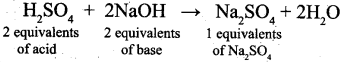Question 2.
Consider the orbitals A, B and C, which as following n and l values,
A ⇒ n = 3 and l = 1
B ⇒ n = 4 and l = 2
C ⇒ n = 2 and l = 0
Arrange the orbitals in increasing energy level.
(a) C < B < A
(b) C < A < B
(c) A < B < C
(d) B < A < C
(b) C < A < BQuestion 3.
The law of triads is obeyed by
(a) Fe, CO, Ni
(b) C, N, O
(c) He, Ne, Ar
(d) Al, Si, P
(a) Fe, CO, Ni

Question 4.
Zeolite used to soften hardness of water is, hydrated
(a) Sodium aluminium silicate
(b) Calcium aluminium silicate
(c) Zinc aluminium borate
(d) Lithium aluminium hydride
Zeolite is sodium aluminium silicate. (NaAlSi2O6 H2O)
(a) Sodium aluminium silicate

Question 5.
Assertion : Generally alkali and alkaline earth metals form superoxides.
Reason : There is a single bond between O and O in superoxides.
(a) both assertion and reason are true and reason is the correct explanation of assertion
(b) both assertion and reason are true but reason is not the correct explanation of assertion
(c) assertion is true but reason is false
(d) both assertion and reason are false
(d) both assertion and reason are falseAmong alkali and alkaline earth metals, K, Rb and Cs alone forms superoxides. Superoxide O2- has 3 electron bond.

Question 6.
Rate of diffusion of a gas is
(a) directly proportional to its density
(b) directly proportional to its molecular weight
(c) directly proportional to its square root of its molecular weight
(d) inversely proportional to the square root of its molecular weight
(d) inversely proportional to the square root of its molecular weight

Question 7.
The values of AH and AS for a reaction are respectively 30 kJ mol-1 and 100 JK 1 mol-1. Then the temperature above which the reaction will become spontaneous is:
(a) 300K
(b) 30K
(c) 100K
(d) 20°C
(a) 300K

∆G = ∆H – T∆S
At 300 K, ∆G = 30000 J mol-1 – 300 K x 100 J K-1 mol-1
∆G = 0 above 300 K ; ΔG will be negative and reaction becomes spontaneous.

Question 8.
If x is the fraction of PCl5 dissociated at equilibrium in the reaction, PCl5 PCl3 + Cl2 then starting with 0.5 mole of PC15, the total number of moles of reactants and products at equilibrium is ……………………… .
(a) 0.5 – x
(b) x + 0.5
(c) 2x + 0.5
(d) x + l
(b) x + 0.5Solution:

 PCl5 PC13 Cl2 Initial no. of moles 0.5 – – No . of moles dissociated X – – No. of moles at equilibrium 0.5 – x X x

Total no. of moles at equilibrium = 0.5 – x + x + x = 0.5 + x

Question 9.
Which of the following concentration terms is/are independent of temperature?
(a) molality
(b) molarity
(c) mole fraction
(d) (a) and (c)
(d) (a) and (c)Solution:
Molality and mole fraction are independent of temperature.

Question 10.
Which one of the following is a correct set?
(a) H2O, sp3, bent
(b) H2O, sp2, linear
(c) NH44+, dsp2, square planar
(d) CH4, dsp2, tetrahedral
(a) H2O, sp3, bent

Question 11.
The IUPAC name of the compound is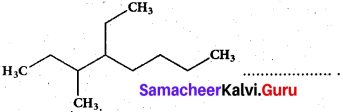(a) 2,3 – Diemethylheptane
(b) 3- Methyl -4- ethyloctane
(c) 5-ethyl -6-methyl octane
(d) 4- Ethyl -3 – methyloctane
(d) 4- Ethyl -3 – methyloctane

Question 12.
Which of the following is correct order of the stability of carbocations?
(a) +CH3 > +CH2 CH2 > +CH(CH3)2 > +C(CH3)3
(b) +CH2CH3 > +CH3 > +CH(CH3)2 > +C(CH3)3
(c) +C(CH3)3 > +CH(CH3)2 > +CH2CH3 > +CH3
(d) +CH(CH3)2 > +CH3 > +CH2CH3 > +C(CH3)3
(c) +C(CH3)3 > +CH(CH3)2 > +CH2CH3 > +CH3Question 13.
where A is,
(a) Zn
(b) Cone. H2SO4
(c) Ale. KOH
(d) Dil. H2SO4
(c) Ale. KOH

Question 14.
Match the compounds given in Column I with suitable items given in Column II.

 Column I (Compound) Column II (Uses) A. Iodoform 1. Fire extinguisher B. Carbon tetrachloride 2. Insecticide C. CFC 3. Antiseptic D. DDT 4. Refrigerants

Code:
(a) A → 2, B → 4, C → 1, D → 3
(b) A → 3, B → 2, C → M, D → 1
(c) A → 1, B → 2, C → 3, D → 4
(d) A → 3, B → 1, C → 4, D → 2
(d) A → 3, B → 1, C → 4, D → 2Question 15.
Living in the atmosphere of CO is dangerous because it …………………………… .
(a) Combines with O2 present inside to form CO2
(b) Reduces organic matter of tissues
(c) Combines with haemoglobin and makes it incapable to absorb oxygen
(d) Dries up the blood
(c) Combines with haemoglobin and makes it incapable to absorb oxygen

Part-II

Answer any six questions in which question No. 20 is compulsory. [6 x 2 = 12]

Question 16.
What is meant by plasma state? Give an example.
The gaseous state of matter at very high temperature containing gaseous ions and free-electron is referred to as the Plasma state, e.g. Lightning.

Question 17.
Give the electronic configuration of Mn2+ and Cr3+.
Mn (Z = 25) Mn → Mn2+ + 2e
Mn2+ electronic configuration is Is2 2s2 2p6 3s2 3p6 3d5
Cr (Z = 24) Cr → Cr3+ + 3e
Cr3+ electronic configuration is Is2 2s2 2p6 3s2 3p6 3d3Question 18.
Do you think that heavy water can be used for drinking purposes?

1. Heavy water (D2O) contains a proton and a neutron. This makes deuterium about twice as heavy as protium, but it is not radioactive. So heavy water is not radioactive.
2. If you drink heavy water, you don’t need to worry about radiation poisoning. But it is not completely safe to drink, because the biochemical reaction in our cells are affected by the difference in the mass of hydrogen atoms.
3. If you drink an appreciable volume of heavy water, you might feel dizzy because of the density difference. It would change the density of the fluid in your inner ear. So it is unlikely to drink heavy water.

Question 19.
Mention the methods used for liquefaction of gases.

1. Linde’s method: Joule-Thomson effect is used to get liquid air or any other gas.
2. Claude’s process: In addition to Joule-Thomson effect, the gas is allowed to perform mechanical work so that more cooling is produced.
3. Adiabatic process: This method of cooling is produced by removing the magnetic property of magnetic material e.g. Gadolinium sulphate. By this method, a temperature of 10-4 K i.e. as low as zero Kelvin can be achieved.

Question 20.
Calculate the entropy change of a process, possessing ΔHt = 2090 J mole-1. Sn(α, 13°C) = Sn(β, 13°C).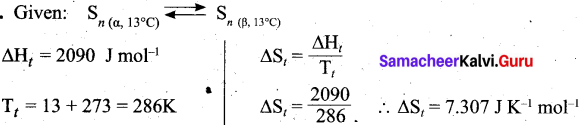Question 21.
What is reaction quotient?
Consider a.homogeneous reversible reaction
For the above reaction under non-equilibrium conditions, reaction quotient Q is defined as the ratio of the product of active masses of reaction products raised to the respective stoichiometric coefficients in the balanced chemical equation to that of the reactants.

Under non equilibrium conditions,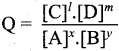Question 22.
Write the structural formula for the following compounds,
(a) Cyclohexa-1,4-diene
(b) Ethynyl Cyclohexane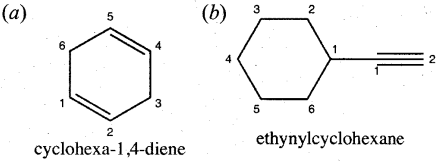Question 23.
What are free radical initiators? Give an example.
The types of reagents that promote homolytic cleavage in substrate are called as free radical initiators. They are short lived and are highly reactive.Example:

• Azobisisobutyronitrile (AIBN)
• Benzoyl peroxide

Question 24.
Complete the reaction and mention name of the reaction.
CH3CH2Br + (CH3)2 LiCu → ?
This reaction is known as Corey-House Mechanism.

Part-III

Answer any six questions in which question No. 29 is compulsory. [6 x 3 = 18]

Question 25.
A compound contains 50% of X (atomic mass 10) and 50% Y (atomic mass 20). Give its empirical formula.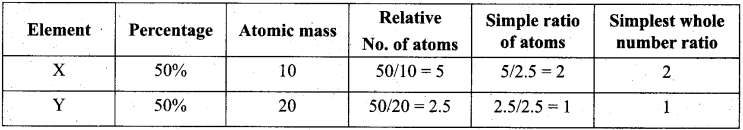∴ The Empirical Formula is X2Y
Empirical Formula mass = 20 + 20 = 40
Molecular mass = Sum of atomic mass = 40
n = 1, Molecular formula = (Empirical Formula )n = (X2Y)1 = X2Y.

Question 26.
Explain Davisson and termer experiment.

• The wave nature of electron was experimentally confirmed by Davisson and Germer.
• They allowed the accelerated beam of electrons to fall on a nickel crystal and recorded the diffraction pattern.
• The resultant diffraction pattern is similar to the X-ray diffraction pattern.
• The finding of wave nature of electron leads to the development of various experimental techniques such as electron microscope, low energy electron diffraction etc.Question 27.
What are the importance of hydrogen bonding in proteins?

• Hydrogen bonds occur in complex biomolecules such as proteins and in biological systems.
• For example, hydrogen bonds play an important role in the structure of deoxyribonucleic acid (DNA), since it holds together the two helical nucleic acid chains.
• In these systems, hydrogen bonds are formed between specific pairs, for example, with a thymine unit in one chain bonding to an adenine unit in another; similarly, a cytosine unit in one chain bonds to a guanine unit in another.
• Intramolecular hydrogen bonding also plays an important role in the structure of polymers, both synthetic and natural.

Question 28.
What is the reason behind the cause of ear pain while climbing a mountain? How it can be rectified?

• When one ascends a mountain in a plain, the external pressure drops while the pressure within the air cavities remains the same. This creates an imbalance.
• The greater internal pressure forces the eardrum to bulge outward causing pain.
• With time and with the help of a yawn or two, the excess air within your ear’s cavities escapes thereby equalizing the internal and external pressure and relieving the pain.Question 29.
A mixture of gases contains 4.76 mole of Ne, 0.74 mole of Ar and 2.5 mole of Xe. Calculate the partial pressure of gases, if the total pressure is 2 atm, at a fixed temperature.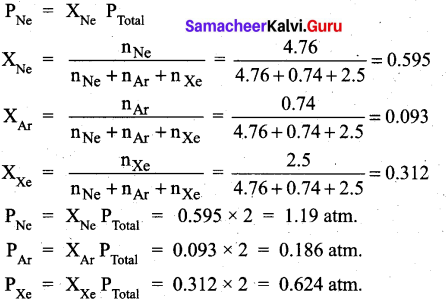Question 30.
Write the various definition of first law of thermodynamics.
The first law of thermodynamics states that “the total energy of an isolated system remains constant though it may change from one form to another” (or) Energy can neither be created nor destroyed, but may be converted from one form to another.Question 31.
How will you detect nitrogen from organic compounds?
Detection of Nitrogen: The following reactions are involved in the detection of nitrogen with formation of prussian blue precipitate conforming the presence of nitrogen in an organic compound.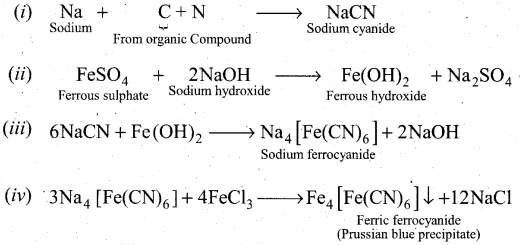Question 32.
Distinguish between carbocation and carbanion.

 Carbocation Carbanion In a carbocation carbon bearing positive charge. In a carbanion carbon bearing negative charge. Carbon bearing positive charge has sp2 hybridization. Carbon bearing negative charge has sp3 hybridization. It has a planar structure. It has a pyramidal structure. E.g.: CH3+, (CH3)2CH, (CH3)3C+etc. E.g.: CH3-CH2, CH3-CHO, (CH3)2CH etc.

Question 33.
What are particulate pollutants? Explain any three.

1. Particulate pollutants are small solid particles, and liquid droplets suspended in air. Examples: dust, pollen, smoke, soot and liquid aerosols.
2. Types of Particulates: Particulates in the atmosphere may be of two types:
• viable particulate and
• non-viable particulate.
3. The viable particulates are small size living organisms such as bacteria, fungi moulds and algae which are dispersed in air.
4. The non-viable particulates are small solid particles and liquid droplets suspended in air. There are four types of non-viable particulates in the atmosphere. They are
(a) Smoke (b) Dust (c) Mist (d) Fumes
5. Smoke: Smoke particulate consists of solid particles formed by combustion of organic matter. For example, cigarette smoke, oil smoke, smokes from burning of fossil fuels, garbage arid dry leaves.
6. Dust: ft is composed of fine solid particles produced during crushing and grinding of solid materials. For example, sand from sand blasting, saw dust from wood works and fly ash from power generating units.
7. Mist: They are formed by particles of sprayed liquids and condensation of vapours in air. For example, sulphuric acid mist, herbicides and insecticides sprays can form mists.
8. Fumes: They are obtained by condensation of vapours released during sublimation, distillation, boiling arid calcination and by several other chemical reactions. For example, organic solvents, metals and metallic oxides.Part – IV

Answer all the questions. [5 x 5 = 25]

Question 34.
(a) (i) The stabilization of a half-filled d-orbital is more pronounced than that of the p-orbital. Why? (3)
(ii) What are degenerate orbitals? (2)
[OR]
(b) (i) Explain the preparation of hydrogen using electrolysis. (3)
(ii) Why hydrogen gas is used as fuel? (2)
(a) (i) The exactly half-filled orbitals have greater stability. The reason for their stability are.
1. symmetry
2. exchange energy.

1. Symmetry: The half filled orbitals are more symmetrical than partially filled orbitals and this symmetry leads to greater stability.

2. Exchange energy: The electrons with same spin in the different orbitals of the same subshell can exchange their position. Each such exchange release energy and this is known as exchange energy. Greater the number of exchanges, greater the exchange energy and hence greater the stability.

In d-orbital, 10 exchanges are possible but in p-orbital 6 exchanges are possible. So, d-orbital with 5 unpaired electrons (10 exchanges)n i.e. half filled is more stable than p-orbital with 3 unpaired electrons (6 exchanges).(ii)

• Three different orientations in space that are possible for a p-orbital. All the three p-orbitals, namely px, py and pz have same energies and are called degenerate orbitals.
• In the presence of magnetic or electric field, the degeneracy is lost.

[OR]

(b) (i) High purity of hydrogen (>99.9%) is obtained by the electrolysis of water containing traces of acid or alkali or electrolysis of aqueous solution of sodium hydroxide or potassium hydroxide using a nickel anode and iron cathode. This process is not economical for large scale production.

At anode: 2OH → H2O + ½ O2 + 2e
At cathode: 2H2O + 2e → 2OH + H2
Overall reaction: H2O → H2 + ½ O2

(ii) Hydrogen bums in air, virtually free from pollution and produces significant amount of energy. This reaction is used in fuel cells to generate electricity.
2H2(g) + O2(g) → 2H2 O(l) + energyQuestion 35.
(a) (i) What is lattice energy? (2)
(ii) Write down the Born-Haber cycle for the formation of CaCl2. (3)
[OR]
(b) (i) What is the effect of added inert gas on the reaction at equilibrium? (2)
(ii) Explain the equilibrium constants for heterogeneous equilibrium. (3)
(a) (i) Lattice energy is defined as the amount of energy required to completely separate one mole of a solid ionic compound into gaseous constituent.
(ii) Born-Haber cycle for the formation of CaCl2.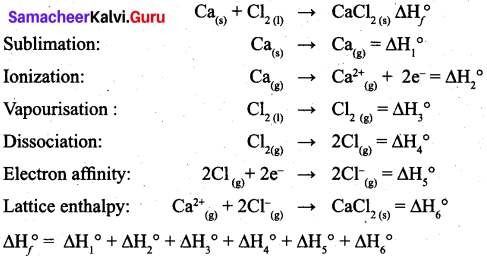[OR]

(b) (i) When an inert gas (i.e., a gas which does not react with any other species involved in equilibrium) is added to an equilibrium system at constant volume, the total number of moles of gases present in the container increases, that is, the total pressure of gases increases, the partial pressure of the reactants and the products are unchanged. Hence at constant volume, the addition of inert gas has no effect on the equilibrium.
(ii) Equilibrium constants for heterogeneous equilibrium:
Consider the following heterogeneous equilibrium.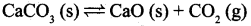The equilibrium constant for the above reaction can be written as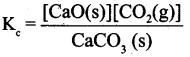A pure solid always has the same concentration at a given temperature, as it does not expand to fill its container, i.e. it has same number of moles L-l of its volume. Therefore, the concentration of a pure solid is constant. The above expression can be modified as follows.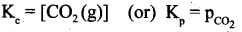The equilibrium constant for the above reaction depends only the concentration of carbon dioxide and pot the calcium carbonate or calcium oxide. Similarly, the active mass (concentration) of the pure liquid does not change at a given temperature. Consequently, the concentration terms of pure liquids can also be excluded from the expression of the equilibrium constant.

For example,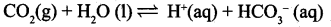Since, H2O (1) is a pure liquid the K can be expressed as $$\mathrm{K}_{\mathrm{c}}=\frac{\left[\mathrm{H}^{+}(\mathrm{aq})\right]\left[\mathrm{HCO}_{3}^{-}(\mathrm{aq})\right]}{\left[\mathrm{CO}_{2}(\mathrm{g})\right]}$$

Question 36.
(a) (i) Explain why the aquatic species are more comfortable in cold water during winter season rather than warm water during the summer? (3)
(ii) What is osmosis? (2)[OR]
(b) (i) Explain the shape of following molecule by using VSEPR theory. (3)
(a) BeCl2 (b) NH3 (c) H2O
(ii) Which bond is stronger or σ or π? Why?
(a)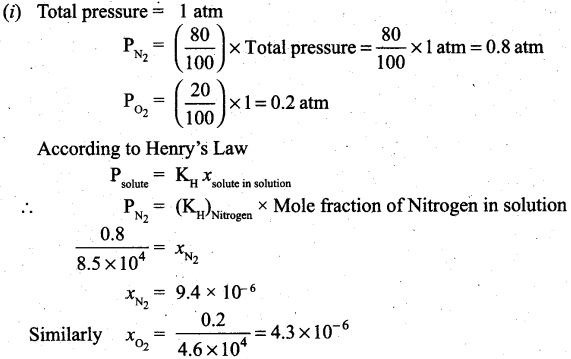(ii) Osmosis is a spontaneous process by which the solvent molecules pass through a semipermeable membrane from a solution of lower concentration to the solution of higher concentration.
[OR]
(b) (i)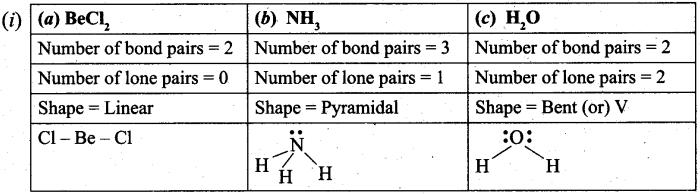(ii)

• Sigma bonds (σ) are stronger than Pi bonds (π). Because, sigma bonds are formed from bonding orbitals directly between the nuclei of the bonding atoms, resulting in greater overlap and a strong sigma bond (axial overlapping).
• Pi bonds results from overlap of atomic orbitals that are in contact through two areas of overlap (lateral overlapping). Pi bonds are more diffused bonds than sigma bonds.Question 37.
(a) (i) How inductive effect helps to explain reactivity and acidity of carboxylic acids? (3)
(ii) HCOOH is more acidic than CH3COOH. Why? (2)
[OR]
(b) (i) Suggest a simple chemical test to distinguish propane and propene. (2)
(ii) Write a notes on Wurtz-fitting reaction. (3)
(a) (i) Reactivity of carboxylic acid:

• When a highly electronegative atom such as halogen is attached to a carbon then it makes the C-X bond polar.
• In such cases the -I effect of halogen facilitates the attack of an incoming nucleophile at the polarized carbon and hence increases the reactivity.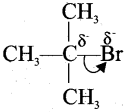• If a -I group is attacher neared to a carbonyl carbon, it decreases the availability of electron density on the carbonyl carbon and hence increases the rate of the nucleophilic addition reaction.Acidity of carboxylic acid:
1. When a halogen atom is attached to the carbon which is neared to the carboxylic acid group, its -I effect withdraws the bonded electrons towards itself and makes the ionization of H+ easy.
2. The acidity of various chloro acetic acid is in the following order.
Cl3C –  COOH > Cl2CHCOOH > CICH2COOH. The strength of the acid increases with increase in the -effect of the group attached to the carboxyl group.
3. Similarly the following order of acidity in the carboxylic acids is due to the +1 effect of alkyl group.
(CH3)3CCOOH < (CH3)2 CHCOOH < CH3COOH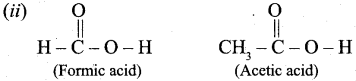Out of Acetic acid and Formic acid, Formic acid is conidered stronger because, CH3 group in Acetic acid contriubutes electron density towards the O – H bond, making it harder to remove the H+ ion and making Acetic acid a weaker acid that Formic acid.

∴ Formic acid is more acidic one.

[OR](b) (i) Chemical test to distinguish between propane and propene
Bromine water test: Propene contains double bond, therefore when we pour the bromine water to propene sample, it decolourises the bromine water whereas propane which is a saturated hydrocarbon does not decolourise the bromine water.
Baeyer’s test: When propene reacts with Bayer’s reagent it gives 1,2 dihydroxy propane. Propane does not react with Baeyer’s reagent.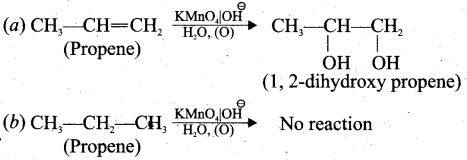(ii) When a solution of bromobenzene and iodomethane in dry ether is treated with metallic sodium, toluene is formed.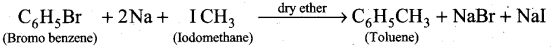Question 38.
(a) An organic compound (A) with molecular formula C2H5Cl reacts with KOH gives compound B and with alcoholic KOH gives compound C. Identify A, B and C explain the reactions. (5)
(b) Write an essay on water pollution. (5)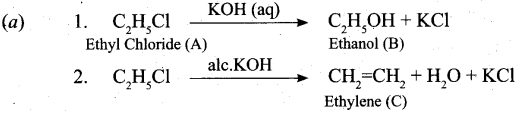[OR]
I – Water Pollution:

1. Water is essential for life. The slogan “Save water, water will save you”, tell us the importance of water.
2. Water pollution is defined as ‘The addition of foreign substances or factors like heat which degrades the quality of water, so that it becomes health hazard or unfit to use.
3. The source of water pollution is classified as point and non-point source. Easily identified source of place of pollution is called as point source, e.g. Municipal and industrial discharge pipes. Non-point source cannot be identified easily, e.g. Acid rain and mining wastes.

II – Causes of water pollution:

• Microbiological (Pathogens): Disease-causing micro organisms like bacteria, viruses and protozoa are most serious water pollutants. They come from domestic sewage and animal excreta.
• Organic wastes: Organic matter such as leaves, grass, trash etc. can also pollute water. Water pollution is caused by excessive phytoplankton growth within water. Micro organisms present in water decompose these organic matter and consume dissolved oxygen in water.
• Chemical wastes: A whole variety of chemicals from industries, such as metals and solvents are poisonous to fish and other aquatic life. Some toxic pesticides can accumulate in fish and shell fish and poison the people who eat them.Harmful effects of chemical water pollutants.

• Cd and Hg can cause kidney damage.
• Pb – poisoning can lead to severe damage of kidneys, liver, brain etc. It also affect central nervous system.
• Poly-chlorinated bipbenol causes skin diseases and are carcinogenic in nature.

III – Quality of drinking water:
Now a days most of us hesitate to use natural water directly for drinking because biological, physical or chemical impurities from different sources mix with surface water or ground water.

Institutes like WHO, BIS and ICMR have prescribed standards for quality of drinking water. Standard characteristics prescribed for deciding the quality of drinking water by BIS, in 1991 are shown in below table.

 Characteristics Desirable limit Physico-chemical Characteristics pH 6.5 to 8.5 Total Dissolved Solids (TDS) 500 ppm Total Hardness (as CaC03) 300 ppm Nitrate 45 ppm Chloride 250 ppm Sulphate 200 ppm Fluoride 1 ppm Biological Characteristics Escherichia Coli (E.Coli) Not at all Coliforms Not to exceed 10 (In 100 ml water sample)